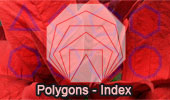# Polygons, Theorems and Problems - Table of ContentThe Base of the Statue of Liberty, Star Polygon of 11 Vertices and the Golden Rectangle.

Polygon Classification. Interactive Mind Map.

Triangles. Theorems and Problems

Pentagons. Theorems and Problems

Hexagons. Theorems and Problems

Heptagons. Theorems and Problems

Octagons. Theorems and Problems

Nonagon or Enneagon. Theorems and Problems

Decagon. Theorems and Problems

Regular Decagon Art.

Optical Prism with Regular Polygonal Form.
Scene created using Prism HD for iPad.

Problem 225. Viviani's Theorem Extension, Regular Polygon, Apothem, Distance.Snowflakes: Hexagonal Symmetry and the Golden Rectangle.
The individual ice crystals often have hexagonal symmetry.Engineering the Impossible: Chartres Cathedral
Circle, Square, Triangle.

Looted Peru Moche Headdress Recovered in London
Octagon: F
eline sea god with octopus-like tentacles

Euclid's Elements Book I, 23 Definitions. One-page visual illustration.
Euclid's Elements Book. Index

Geometry Problem 1430.
Regular Octagon, Area, Equivalent Figures, Poster, Conformal Mapping.

Geometry Problem 1357.
Regular Dodecagon, Diagonals, Concurrency, Collinearity, Points, Lines.

Geometry Problem 1325.
Octagons, Midpoints, Squares, Mobile Apps, iPad.

Geometry Problem 1313.
Regular Decagon, Pentadecagon, Equilateral Triangle, Congruence.

Geometry Problem 725.
Kurschak's Dodecagon, Square, Equilateral Triangle, Polygon, Midpoints, 30, 60 Degrees.

Proposed Problem 430.
Circumscribed and Inscribed Regular Pentagon, Perpendicular, Areas.

 Home | Sitemap | Search | Geometry | Post a comment | Email | by Antonio Gutierrez Last updated: Mar 30, 2019